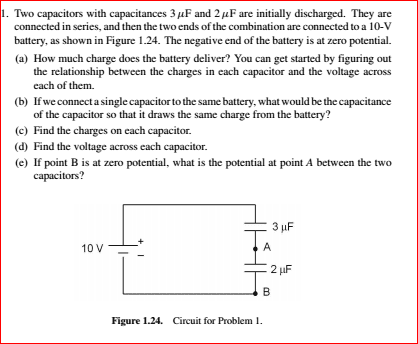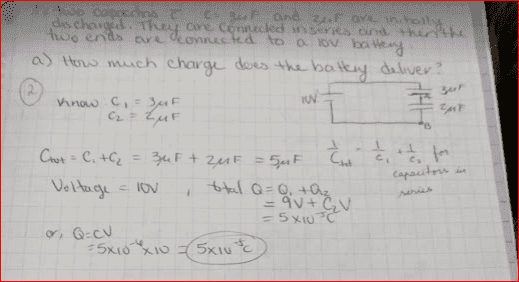# How much charge does the battery deliver?

## Homework Statement## Homework Equations

/ 3. The Attempt at a Solution [/B]
Here is what I attempted for the HW. I only got 2/10 for this here so I'm wanting to correct my mistakes.cnh1995
Homework Helper
Gold Member
How do you get the equivalent capacitance of capacitors in series?

1/Ceq = 1/C1 + 1/C2 = 5/6mF

cnh1995
Homework Helper
Gold Member
1/Ceq = 1/C1 + 1/C2 = 5/6mF
It's 6/5 mF (you forgot to take the reciprocal).

So then the circuit becomes one with one capacitor Ceq= 5/6mF
And we know Q=CV
So, Q=(5/6mF)(10V) = 25/3 mC = 8.33x10^-6F?

It's 6/5 mF (you forgot to take the reciprocal).
Whoops! So I did.
So Ceq=6/5mF and then plug that into the Q=CV equation?

Ceq = 1/(1/C1 + 1/C2) = C1*C2/(C1+C2)

nevermind, you picked it up, and I can't delete this

cnh1995
Homework Helper
Gold Member
Whoops! So I did.
So Ceq=6/5mF and then plug that into the Q=CV equation?
Yes.

epenguin
Homework Helper
Gold Member
I see that in at least two problems in a short interval you make a similar stumbles, though you finally get it right. But since you seem to think in formulae and you forget them, even if you get it right now in six or 12 months time when you need it you're likely have forgotten and could stumble again. Cure IMO is to think physically not formulaically.

Physically, in the nature of what voltage is, voltages across elements in series add up (be the elements resistors, capacitors or inductors). Whereas for elements in parallel the voltage across them is the same for each almost by definition. By the nature of charge electric charge, the charge on capacitors in parallel add up to total charge, while capacitors in series each have the same charge.

I blame a lot of stumbles of students on the misleading slangy phrase "charge on a capacitor". Creating a charge - well actually you can't create any - but separating electrical charges is energetically expensive. So a capacitor does not have a charge. What it has is a charge separation – equal and opposite positive and negative charges close to each other, typically only a micrometer distant from each other, so overall neutrality. Known, elementary and obvious, maybe but I think students need to actively remind themselves when doing problems in order not to get confused.

•